# Functional Reactive Programming

## What is FRP?

$$\newcommand{\lb}{\unicode{x27E6}}$$ $$\newcommand{\rb}{\unicode{x27E7}}$$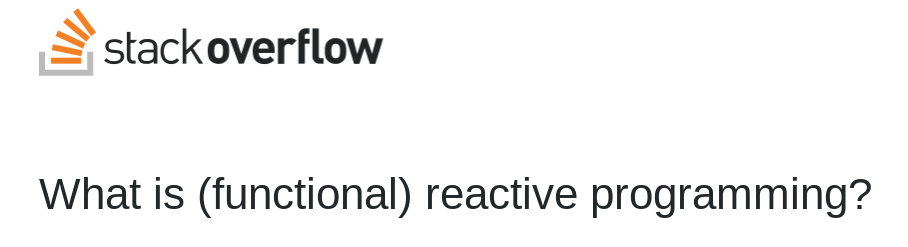## FRP is abstract

• what it is
• definition
• why it's interesting
• synthesis

## What is it?

• programming with time-varying values
• time is an explicit part of the programming model
• composable, declarative

## What it is

• Behavior: changes continuously
• mouse position: Behavior (Int, Int)
• Event: happens at a point in time
• keyboard press: Event Char

## Behavior vs Event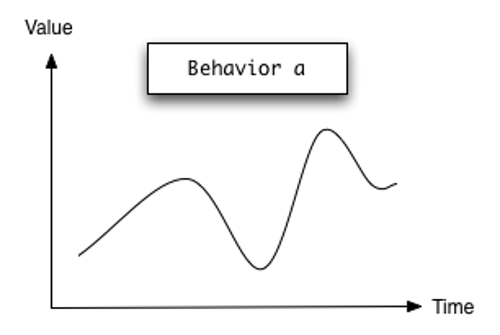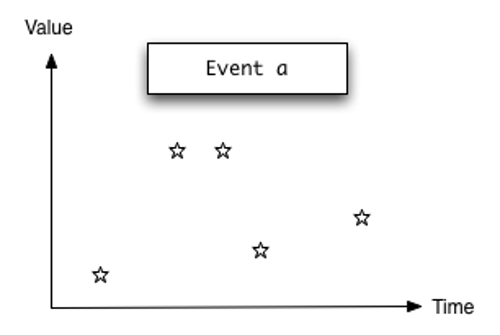(From reactive-banana documentation.)

## Declarative: Simple Semantics

• Time $$T$$
• $$\lb\text{Behavior}\ a\rb = T \to a$$
• $$\lb\text{Event}\ a\rb = (T, a)$$
• $$\text{Stream}\ a = \text{Event}(a, \text{Stream}\ a)$$
• first-class values

## Temporal Logic

• $$\square P(x)$$: $$P(x)$$ always holds
• $$\Diamond P(x)$$: $$P(x)$$ eventually holds
• Symmetrical:
• $$\square P(x) \Leftrightarrow \lnot\Diamond\lnot P(x)$$
• $$\Diamond P(x) \Leftrightarrow \lnot\square\lnot P(x)$$

## Curry-Howard

• $$\text{Behavior}\ a : \square a$$
• $$\text{Event}\ a\hspace{1.3em}: \Diamond a$$
• symmetrical!

## Generalities:

• abstract types:
• Behavior a
• Event a
• no literal “time” values

## Inputs

mouse    :: Behavior (Int, Int)
keypress :: Event KeyCode
click    :: Event (Int, Int)

data TextWidget = {
text  :: Behavior String
typed :: Event ()
}

-- robotics
camera :: Behavior Image
bump   :: Event ()


## Combinators

when  :: B Bool -> E a -> E a
at    :: B a -> E b -> E a
union :: E a -> E a -> E a
steps :: E a -> B a
foldP :: (a -> b -> b) -> E a -> E b

• Functor, Applicative, Monoid… etc

## Output

set :: Element -> Attribute a ->
B a -> IO ()
handle :: E a -> (a -> IO ()) -> IO ()


## Life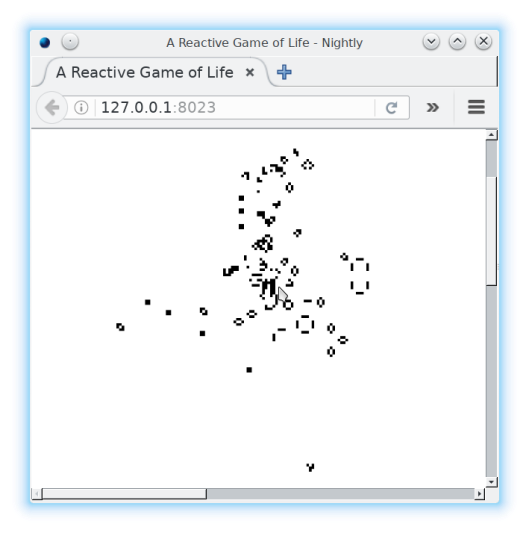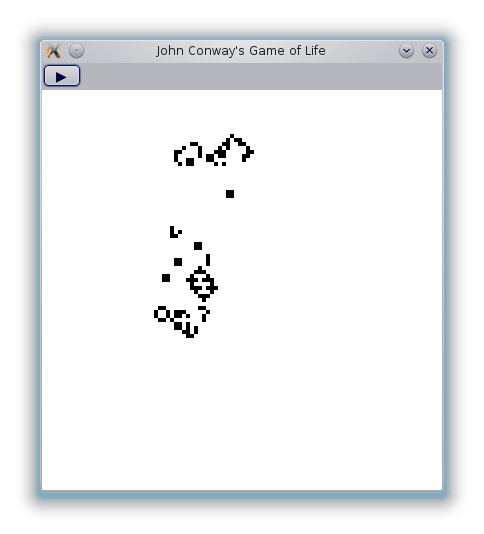Game code:

blank :: Int -> Int -> Grid
rPentonimo :: Grid
step :: Grid -> Grid
modify :: (Int, Int) -> Grid -> Grid

• Widgets:
• canvas: contains game of life
• pauseButton: pauses animation
• timer: sends an event every 200 milliseconds

## Input

-- every 200ms from timer
ticks :: Event ()

mouse :: Behavior Point
click :: Event ()

-- button presses
pauses :: Event ()


## Operators:

f $x = f x f <$> xs = fmap f xs

x' <$xs = fmap (const x') xs () <$ [1,2,3,4] = [(), (), (), ()]


## Combinators

active <- accumB False (not <$pauses) steps, modifies :: Event (Grid -> Grid) steps = whenE active (step <$ ticks)
modifies = modify . adjust <$> clicks changes = updates union modifies life <- accumE start changes  ## Output • redraw canvas on change • depends on II framework ## Adding Features generation <- accumB 0 ((+ 1) <$ steps)

• no changing old code
• very modular

## Libraries:

• reactive-banana
• fast, good semantics
• threepenny-gui:
• lightweight UI framework
• prototyping, internal tools
• FRP layers based on reactive-banana

## Libraries

• reflex-frp
• fast, good semantics
• integrates with GHCJS, DOM
• used in production at Skedge.me?
• easy install: reflex-platform
• builds GHCJS/reflex using Nix

## Open Questions

• FRP is an active research field
• performance optimization
• correctness
• nested events/behaviors
• think TODO MVC
• organizing larger programs

## Open Questions

• dependent typing
• $$a \triangleright b$$: $$a$$ until $$b$$
• alternative to Haskell IO type